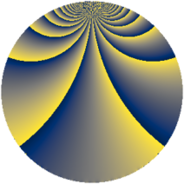# Properties

 Label 552.2.jLevel $552$ Weight $2$ Character orbit 552.j Rep. character $\chi_{552}(323,\cdot)$ Character field $\Q$ Dimension $88$ Newform subspaces $4$ Sturm bound $192$ Trace bound $5$

# Related objects

## Defining parameters

 Level: $$N$$ $$=$$ $$552 = 2^{3} \cdot 3 \cdot 23$$ Weight: $$k$$ $$=$$ $$2$$ Character orbit: $$[\chi]$$ $$=$$ 552.j (of order $$2$$ and degree $$1$$) Character conductor: $$\operatorname{cond}(\chi)$$ $$=$$ $$24$$ Character field: $$\Q$$ Newform subspaces: $$4$$ Sturm bound: $$192$$ Trace bound: $$5$$ Distinguishing $$T_p$$: $$5$$

## Dimensions

The following table gives the dimensions of various subspaces of $$M_{2}(552, [\chi])$$.

Total New Old
Modular forms 100 88 12
Cusp forms 92 88 4
Eisenstein series 8 0 8

## Trace form

 $$88q - 3q^{6} + O(q^{10})$$ $$88q - 3q^{6} - 8q^{10} - 13q^{12} - 8q^{16} + 21q^{18} + 4q^{22} + 20q^{24} + 88q^{25} - 12q^{27} + 12q^{28} - 22q^{30} - 8q^{33} + 12q^{34} - q^{36} + 8q^{40} - 8q^{42} + 32q^{43} + 5q^{48} - 104q^{49} + 40q^{51} - 2q^{52} + 20q^{54} - 8q^{57} - 42q^{58} - 24q^{60} - 18q^{64} - 14q^{66} + 48q^{70} - 72q^{72} - 16q^{73} - 56q^{75} + 44q^{76} - 35q^{78} + 8q^{81} + 54q^{82} - 14q^{84} - 12q^{88} - 46q^{90} + 48q^{91} + 2q^{94} - 77q^{96} + 32q^{97} + 64q^{99} + O(q^{100})$$

## Decomposition of $$S_{2}^{\mathrm{new}}(552, [\chi])$$ into newform subspaces

Label Dim. $$A$$ Field CM Traces $q$-expansion
$$a_2$$ $$a_3$$ $$a_5$$ $$a_7$$
552.2.j.a $$2$$ $$4.408$$ $$\Q(\sqrt{-2})$$ None $$0$$ $$-2$$ $$-8$$ $$0$$ $$q+\beta q^{2}+(-1+\beta )q^{3}-2q^{4}-4q^{5}+\cdots$$
552.2.j.b $$2$$ $$4.408$$ $$\Q(\sqrt{-2})$$ None $$0$$ $$-2$$ $$8$$ $$0$$ $$q+\beta q^{2}+(-1+\beta )q^{3}-2q^{4}+4q^{5}+\cdots$$
552.2.j.c $$42$$ $$4.408$$ None $$0$$ $$2$$ $$-8$$ $$0$$
552.2.j.d $$42$$ $$4.408$$ None $$0$$ $$2$$ $$8$$ $$0$$

## Decomposition of $$S_{2}^{\mathrm{old}}(552, [\chi])$$ into lower level spaces

$$S_{2}^{\mathrm{old}}(552, [\chi]) \cong$$ $$S_{2}^{\mathrm{new}}(24, [\chi])$$$$^{\oplus 2}$$